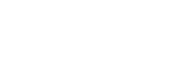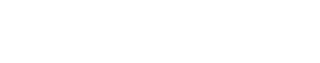Understanding Cognitive Development According to Experts

According to the Content Standards of the Minister of National Education Number 22 of 2006, mathematics begins to be studied in elementary schools, so that students can understand mathematics well it is necessary to understand basic concepts in mathematics. According to the theory of J. Piaget, a person’s cognitive development from infancy to adulthood is divided into four stages:

1. Sensory motor stage (0 – 2 years)
2. Pre-operational stage (2 – 7 years)
3. Concrete operational stage (7 – 11 years)
4. Formal stage (over 11 years old)

Based on Piaget’s theory, it appears that at the beginning, children learn through things that are concrete or real in the sense that they can be observed using their five senses. Therefore, to understand an abstract mathematical concept, children need concrete objects.

Besides Piaget, several other experts expressed their opinion about the development of one’s learning, namely Bruner. Bruner (in Fajar, 2008: 15) divides the student learning process into three stages, namely the enactive, iconic and symbolic stages.

1. Enactive Stage

At this stage, students are required to learn knowledge by using concrete objects or using real situations for students.

2. Iconic Stage

After studying knowledge with real or concrete objects, the next stage is the iconic stage, where students learn knowledge in the form of pictures or diagrams as an embodiment of activities that use concrete or real objects.

3. Symbolic Stage

In addition to the two stages above, there is one more stage, namely the symbolic stage where students manifest their knowledge in the form of abstract symbols. In other words, students must experience the process of abstraction.

According to Bruner, learning should start by using real objects first. Therefore, when the mathematics learning process takes place, it is necessary to use models or real objects for certain topics that can help students understand. Thus, it is clear that teaching aids in learning mathematics are indispensable.

Based on the explanation above, junior high school students are transitioning from the concrete operational stage to the formal stage, so in teaching mathematics to students, demonstrations are still needed so that learning becomes meaningful in increasing students’ understanding and interest in learning mathematics (in Widyantini and Guntoro, 2009: 3-5 ).

Understanding Understanding Concepts

Understanding comes from the word understanding which means a lot of knowledge, understand right, know right. Furthermore, the word “understanding” in the Big Indonesian Dictionary in 2002 understands something correctly (Ministry of National Education, 2002).

According to Womack, the concept can be seen from the connotative and denotative terms. The concept in the dictionary, denotative understanding is an idea or understanding that is abstracted from concrete events. In a connotative sense, the concept has a broader meaning, including:

1. The concept is a collection of abstract meanings (the abstract body of meaning) related to symbols for the class of an object (object) event or idea.
2. Concepts are abstract and contain meanings that relate to all possible class members (not to one particular instance of the class).
3. Concepts are subjective and internalized.

Concept according to Moore is “something stored in the mind-a thought, an idea or an idea”. Meanwhile, Parker stated that “a concept is an idea that exists through examples”.

From the several notions of the concept above, it can be concluded that the concept is an idea in the form of an abstract understanding related to symbols that are internalized from existing examples or from concrete events. Understanding the concept means understanding correctly about the abstract meaning that is internalized from concrete examples or events (Sadi, 2010).

Learning Media

Learning media is a tool that can help students so that the learning process occurs. By using learning media, it is expected that

1. Students can get a variety of real experiences so that learning materials are easy to understand,
2. Can increase student learning motivation,
3. Can encourage students to remember what they have learned.

According to Estiningsih (in Widyantini and Guntoro, 2009: 3) teaching aids are learning media that contain or convey the characteristics of the concepts being studied. The main function of teaching aids is to reduce the abstraction of the concept, so that students are able to understand the meaning of the concept. By seeing, feeling, manipulating objects/props, students have experience in everyday life about the meaning of a concept.

Some of the functions of math props are to:

1. Makes it easier to understand a concept in mathematics,
2. Reinforce or display the concepts that have been given,
3. Motivate or arouse students’ interest in a concept,
4. Learning Resources.

Posinega Card

The Posinega Card is a demonstration tool for integer addition and subtraction operations consisting of cards with a positive (+) sign and cards with a negative sign (–). This posinega card is used to help students understand the concept of adding and subtracting integers.

Some of the provisions that must be agreed upon in demonstrating the operation of addition and subtraction of integers on this teaching aid are:

a. Provide cards marked ” + ” and cards marked ” – “, a minimum of 20 cards each. Cards marked “+ ” are used to represent positive integers and cards marked “– ” are used to represent negative integers.

b. The addition operation is the process of adding/giving a “+” or “–” card. While the subtraction operation is the process of taking a “+” card or a “–” card.

The rules are:c. If a card with a positive sign meets (paired with a card with a negative sign, the result is zero.Posinega Card Usage

1. The use of posinega cards in integer addition operations

a) Determine the result 2 + 3 = …

Place two “+” cards to represent the number 2 in the first term and add three “+” cards to represent the number 3 in the second term, resulting in five “+” cards. From the demonstration, it can be seen that 2 added to 3 produces 5.b) Determine the result of 2 + (-3) = …

The process is identical to the example of part a) namely:c) Determine the result (-2) + 3 = …d) Determine the result (-2) + (-3) = …2. The use of posinega cards in integer subtraction operations

a) Determine the result 3 – 2 = …

Place three cards marked “+” to represent the number 3 in the first term. Next take two cards marked “+” to show minus two and look at the remaining cards. From this demonstration, the result of subtraction 3 – 2 = 1.b) Determine the result of 2 – 3 = …

The process is identical to the example of part a) namely:c) Determine the result 2 – (-3) = …d) Determine the result (-2) – (-3) = ….